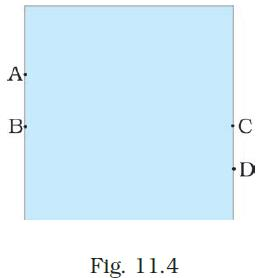Courses

# Test: Force & Pressure- 1

## 10 Questions MCQ Test Science Class 8 | Test: Force & Pressure- 1

Description
This mock test of Test: Force & Pressure- 1 for UPSC helps you for every UPSC entrance exam. This contains 10 Multiple Choice Questions for UPSC Test: Force & Pressure- 1 (mcq) to study with solutions a complete question bank. The solved questions answers in this Test: Force & Pressure- 1 quiz give you a good mix of easy questions and tough questions. UPSC students definitely take this Test: Force & Pressure- 1 exercise for a better result in the exam. You can find other Test: Force & Pressure- 1 extra questions, long questions & short questions for UPSC on EduRev as well by searching above.
QUESTION: 1

### A push or pull on an object is called

Solution:

A push or pull on an object is called force. Force can make stationary body in motion and moving body in rest.

QUESTION: 2

### Which one of the following forces is a contact force?

Solution:

Contact forces are those that result when the two interacting objects are perceived to be physically contacting each other.
Example: Frictional forces, tensional forces, normal forces, air resistance forces, and applied forces.

QUESTION: 3

### S.I. unit of force is

Solution:

S.I. unit of force is Newton.
One Newton is a force required to push a body of mass of one kg to a distance of one meter.

QUESTION: 4

In game of tug-of war, two teams

Solution:

In a game of tug-of war, two teams pull the rope in opposite direction. The team that pulls the rope toward itself will become winner.

QUESTION: 5

Perpendicular force acting per unit area is called

Solution:

The total force acting perpendicular on a given surface is called thrust. Thrust acting upon a unit area is called pressure.

QUESTION: 6

A water tank has four taps fixed at points A, B, C, D as shown in Fig. 11.4. The water will flow out at the same pressure from taps atSolution:

In the given water tank, B and C are at the same levels. Hence, from taps B and C, water will flow out at the same pressure.

QUESTION: 7

The net force on an object is __________ if the two forces acting on it in opposite directions are equal.

Solution:

The force on an object is zero if the two forces acting on it in opposite directions are equal in magnitude. This kind of force is called balanced force.

QUESTION: 8

A force of 16N acts on an area of 50 cm2. What is the pressure in pascal?

Solution:

F = 16 N,
A = 50 cm2 = 50 / 10000 m2
We know that, P = F / A
∴ P = (16 x 10000) / 50 = 3200 Pa

QUESTION: 9

A force applied on an object may change its

Solution:

A force applied on an object may change its speed.

If the force applied on the object is in the direction of its motion, the speed of the object increases.
If the force applied on the object is in the direction opposite to the direction of motion, the speed of the object decreases.

QUESTION: 10

A force of 1200 N acts on the surface of an area 10 cm2 normally. What would be the thrust and pressure on the surface?

Solution:

Force, F = 1200 N
Area, A = 10 cm2 = 10×10-4 m2 = 10-3 m2

Thrust (perpendicular force), F = 1200 N

Pressure, P =  F/A = 1200/10−3 = 1.2×106 N/m2